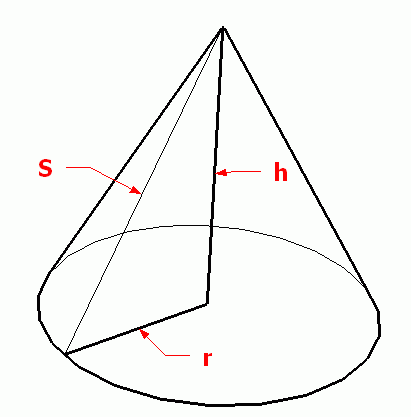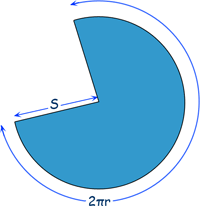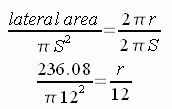SEARCH HOMEMath Central Quandaries & QueriesQuestion from Crystal, a student: In my homework the question says the lateral area of a right cone is 226.08 cm cubed. the slant hieght is 12 cm. Find the total surface area. How do I do that?Hi Crystal.

The lateral area is the surface area of a three dimensional object excluding the area of the base.

So it doesn't make sense to me that the question says 226.08 cm3 , because that is a volume measurement. I am hoping that is a typo and you meant to write 226.08 cm2 .

The base of a right cone is a circle, so if you can find the radius, you can find the area of the circle, add that to the lateral area and you have the total surface area.You are given the slant height, so S = 12 cm.

The lateral surface area of a right circular cone is the same as the area of the sector of the circle it can be unrolled into. Note that the circumference around the bottom edge must be 2πr. The slant length is S, so we have this pattern:The ratio of the area of the blue region to the area of the full circle is equal to the ratios of the arc length of the blue region to the circumference of the full circle. Since you know that the area of the blue region is the lateral area of the cone, you can writeSo you can find r.

Hope this helps,
Stephen La Rocque.Math Central is supported by the University of Regina and The Pacific Institute for the Mathematical Sciences.